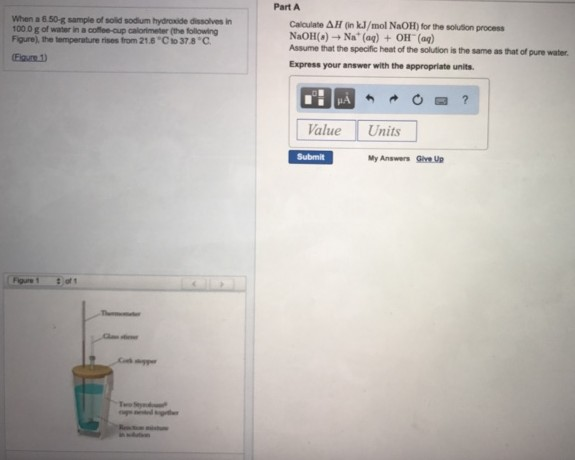# Problem: When a 6.50 g sample of solid sodium hydroxide dissolves in 100.0 g of water in a coffee-cup calorimeter (the following Figure), the temperature rises from 21.6°C to 37.8°C.Calculate ΔH (in kJ/mol NaOH) for the solution process NaOH(g) → Na+(aq) + OH-(aq) Assume that the specific heat of the solution is the same as that of pure water. Express your answer with the appropriate units.

###### FREE Expert Solution
79% (45 ratings)###### Problem Details

When a 6.50 g sample of solid sodium hydroxide dissolves in 100.0 g of water in a coffee-cup calorimeter (the following Figure), the temperature rises from 21.6°C to 37.8°C.

Calculate ΔH (in kJ/mol NaOH) for the solution process

NaOH(g) → Na+(aq) + OH-(aq)

Assume that the specific heat of the solution is the same as that of pure water.